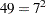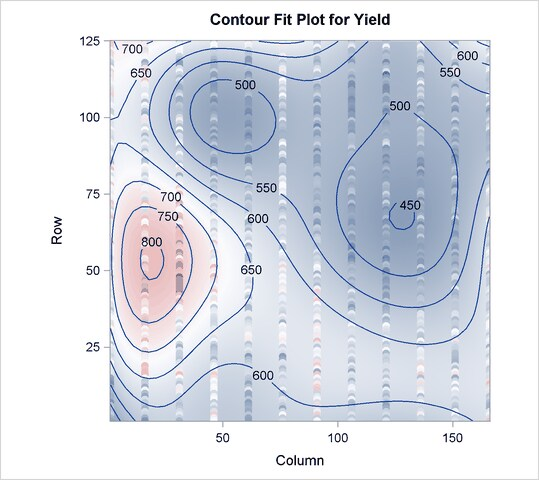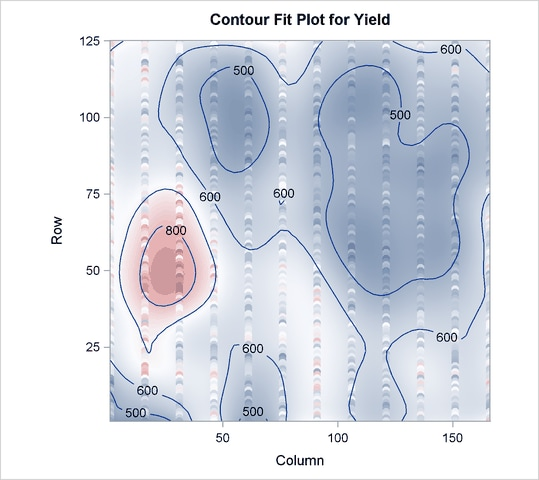## Example 69.4 Partial Least Squares Spline Smoothing

The EFFECT statement makes it easy to construct a wide variety of linear models. In particular, you can use the spline effect to add smoothing terms to a model. A particular benefit of using spline effects in PROC PLS is that, when operating on spline basis functions, the partial least squares algorithm effectively chooses the amount of smoothing automatically, especially if you combine it with cross validation for the selecting the number of factors. This example employs the EFFECT statement to demonstrate partial least squares spline smoothing of agricultural data.

Weibe (1935) presents data from a study of uniformity of wheat yields over a certain rectangular plot of land. The following statements read these wheat yield measurements, indexed by row and column distances, into the SAS data set Wheat:

```data Wheat; keep Row Column Yield;
input Yield @@;
iRow = int((_N_-1)/12);
iCol = mod( _N_-1 ,12);
Column = iCol*15 + 1; /* Column distance, in feet */
Row    = iRow* 1 + 1; /* Row    distance, in feet */
Row = 125 - Row + 1;  /* Invert rows              */
datalines;
715 595 580 580 615 610 540 515 557 665 560 612
770 710 655 675 700 690 565 585 550 574 511 618
760 715 690 690 655 725 665 640 665 705 644 705
665 615 685 555 585 630 550 520 553 616 573 570
755 730 670 580 545 620 580 525 495 565 599 612
745 670 585 560 550 710 590 545 538 587 600 664
645 690 550 520 450 630 535 505 530 536 611 578

... more lines ...

570 585 635 765 550 675 765 620 608 705 677 660
505 500 580 655 470 565 570 555 537 585 589 619
465 430 510 680 460 600 670 615 620 594 616 784
;
```

The following statements use the PLS procedure to smooth these wheat yields using two spline effects, one for rows and another for columns, in addition to their crossproduct. Each spline effect has, by default, seven basis columns; thus their crossproduct hascolumns, for a total of 63 parameters in the full linear model. However, the predictive PLS model does not actually need to have 63 degrees of freedom. Rather, the degree of smoothing is controlled by the number of PLS factors, which in this case is chosen automatically by random subset validation with the CV=RANDOM option.

```ods graphics on;

proc pls data=Wheat cv=random(seed=1) cvtest(seed=12345)
effect splCol = spline(Column);
effect splRow = spline(Row   );
model Yield = splCol|splRow;
run;

ods graphics off;
```

These statements produce the output shown in Output 69.4.1 through Output 69.4.4.

Output 69.4.1 Default Spline Basis: Model and Data Information
The PLS Procedure

Data Set WORK.WHEAT Partial Least Squares NIPALS 1 63 Exclude 15 10-fold Random Subset Validation 1 Prob T**2 > 0.1 1000 12345

 Number of Observations Read 1500 1500

Output 69.4.2 Default Spline Basis: Random Subset Validated PRESS Statistics for Number of Factors
Random Subset Validation for the
Number of Extracted Factors
Number of
Extracted
Factors
Root Mean PRESS T**2 Prob > T**2
0 1.066355 251.8793 <.0001
1 0.826177 123.8161 <.0001
2 0.745877 61.6035 <.0001
3 0.725181 44.99644 <.0001
4 0.701464 23.20199 <.0001
5 0.687164 8.369711 0.0030
6 0.683917 8.775847 0.0010
7 0.677969 2.907019 0.0830
8 0.676423 2.190871 0.1340
9 0.676966 3.191284 0.0600
10 0.675026 1.334638 0.2390
11 0.673906 0.556455 0.4470
12 0.673653 1.257292 0.2790
13 0.672669 0 1.0000
14 0.673596 2.386014 0.1190
15 0.672828 0.02962 0.8820

 Minimum root mean PRESS 0.6727 13 8

Output 69.4.3 Default Spline Basis: PLS Variation Summary for Split-Sample Validated Model
Percent Variation Accounted for by Partial
Least Squares Factors
Number of
Extracted
Factors
Model Effects Dependent Variables
Current Total Current Total
1 11.5269 11.5269 40.2471 40.2471
2 7.2314 18.7583 10.4908 50.7379
3 6.9147 25.6730 2.6523 53.3902
4 3.8433 29.5163 2.8806 56.2708
5 6.4795 35.9958 1.3197 57.5905
6 7.6201 43.6159 1.1700 58.7605
7 7.3214 50.9373 0.7186 59.4790
8 4.8363 55.7736 0.4548 59.9339

Output 69.4.4 Default Spline Basis: Smoothed YieldThe cross validation results in Output 69.4.2 point to a model with eight PLS factors; this is the smallest model whose predicted residual sum of squares (PRESS) is insignificantly different from the model with the absolute minimum PRESS. The variation summary in Output 69.4.3 shows that this model accounts for about 60% of the variation in the Yield values. The OBS=GRADIENT suboption for the PLOT=CONTOURFIT option specifies that the observations in the resulting plot, Output 69.4.4, be colored according to the same scheme as the surface of predicted yield. This coloration enables you to easily tell which observations are above the surface of predicted yield and which are below.

The surface of predicted yield is somewhat smoother than what Weibe (1935) settled on originally, with a predominance of simple, elliptically shaped contours. You can easily specify a potentially more granular model by increasing the number of knots in the spline bases. Even though the more granular model increases the number of predictor parameters, cross validation can still protect you from overfitting the data. The following statements are the same as those shown before, except that the spline effects now have twice as many basis functions:

```ods graphics on;

proc pls data=Wheat cv=random(seed=1) cvtest(seed=12345)
effect splCol = spline(Column / knotmethod=equal(14));
effect splRow = spline(Row    / knotmethod=equal(14));
model Yield = splCol|splRow;
run;

ods graphics off;
```

The resulting output is shown in Output 69.4.5 through Output 69.4.8.

Output 69.4.5 More Granular Spline Basis: Model and Data Information
The PLS Procedure

Data Set WORK.WHEAT Partial Least Squares NIPALS 1 360 Exclude 15 10-fold Random Subset Validation 1 Prob T**2 > 0.1 1000 12345

 Number of Observations Read 1500 1500

Output 69.4.6 More Granular Spline Basis: Random Subset Validated PRESS Statistics for Number of Factors
Random Subset Validation for the
Number of Extracted Factors
Number of
Extracted
Factors
Root Mean PRESS T**2 Prob > T**2
0 1.066355 247.9268 <.0001
1 0.652658 20.68858 <.0001
2 0.615087 0.074822 0.7740
3 0.614128 0 1.0000
4 0.615268 0.197678 0.6490
5 0.618001 1.372038 0.2340
6 0.622949 5.035504 0.0180
7 0.626482 7.296797 0.0080
8 0.633316 13.66045 <.0001
9 0.635239 16.16922 <.0001
10 0.636938 18.02295 <.0001
11 0.636494 16.9881 <.0001
12 0.63682 16.83341 <.0001
13 0.637719 16.74157 <.0001
14 0.637627 15.79342 <.0001
15 0.638431 16.12327 <.0001

 Minimum root mean PRESS 0.6141 3 2

Output 69.4.7 More Granular Spline Basis: PLS Variation Summary for Split-Sample Validated Model
Percent Variation Accounted for by Partial
Least Squares Factors
Number of
Extracted
Factors
Model Effects Dependent Variables
Current Total Current Total
1 1.7967 1.7967 64.7792 64.7792
2 1.3719 3.1687 6.3163 71.0955

Output 69.4.8 More Granular Spline Basis: Smoothed YieldOutput 69.4.5 shows that the model now has 360 parameters, many more than before. In Output 69.4.6 you can see that with more granular spline effects, fewer PLS factors are required—only two, in fact. However, Output 69.4.7 shows that this model now accounts for over 70% of the variation in the Yield values, and the contours of predicted values in Output 69.4.8 are less inclined to be simple elliptical shapes.